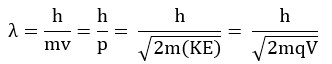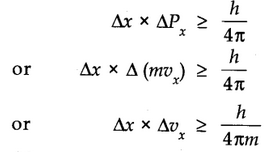# Dual behaviour of matter (de Broglie Equation)

de Broglie proposed that matter should also exhibit dual behaviour i.e. both particle and wave like properties.where p is the linear momentum of a particle.

According to de Broglie, every object in motion has a wave character. The wavelengths associated with ordinary objects are so short (because of their large masses) that their wave properties cannot be detected. The wavelengths associated with electrons and other subatomic particles (with very small mass) can, however, be detected experimentally.

# Heisenberg’s Uncertainty Principle

It states that it is impossible to determine simultaneously, the exact position and exact momentum (or velocity) of an electron.

Mathematically, it can be given as in equation,Where ∆x is the uncertainty in position and ∆Px (or ∆ Vx) is the uncertainty in momentum (or velocity) of the particle and h is Planck’s constant.

## The significance of Uncertainty Principle

• It rules out the existence of definite paths or trajectories of electrons and other similar particles.
• The effect of Heisenberg’s uncertainty principle is significant only for microscopic objects and is negligible for macroscopic objects.

The effect of the Heisenberg Uncertainty Principle is significant only for the motion of microscopic objects and is negligible for that of macroscopic objects.

## Failure of Bohr’s model

• It ignores the dual behaviour of matter.
• It contradicts Heisenberg’s uncertainty principle.